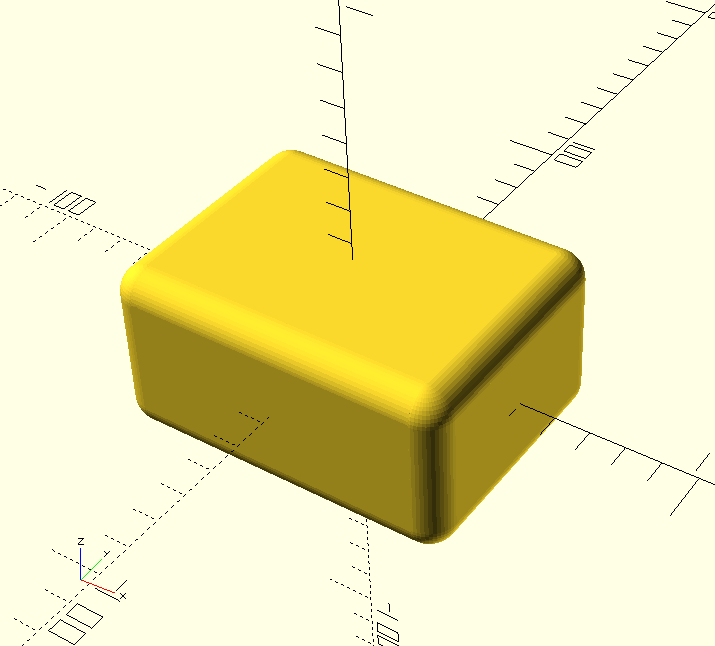Filed Under Programming
posted by sysrpl on Sunday April 21, 2019 10:52 PM

I've been tinkering with building electronics and have needed to fabricate a few parts. A few days ago I found OpenSCAD and as a programmer I love the idea of doing solid object modeling using purely source code to define 3D shapes. OpenSCAD is fairly limited by itself, but if it doesn't do something you can always program it to do what you want. Here is a small OpenSCAD module to render rounded boxes.```module cubed(size = [10, 10, 10], radius = 0, center = true/false)
{
if (radius < 1)
{
cube(size, center);
}
else
{
move = center ? [0, 0, 0] : [size.x / 2, size.y / 2, size.z / 2];
translate(move)
{
union()
{
cube([size.x - radius * 2, size.y - radius * 2, size.z], center = true);
cube([size.x - radius * 2, size.y, size.z - radius * 2], center = true);
cube([size.x, size.y - radius * 2, size.z - radius * 2], center = true);

translate([size.x / -2 + radius, size.y / -2 + radius,
size.z / -2 + radius])
translate([size.x / 2 - radius, size.y / -2 + radius,
size.z / -2 + radius])
translate([size.x / -2 + radius, size.y / 2 - radius,
size.z / -2 + radius])
translate([size.x / 2 - radius, size.y / 2 - radius,
size.z / -2 + radius])

translate([size.x / -2 + radius, size.y / -2 + radius,
size.z / 2 - radius])
translate([size.x / 2 - radius, size.y / -2 + radius,
size.z / 2 - radius])
translate([size.x / -2 + radius, size.y / 2 - radius,
size.z / 2 - radius])
translate([size.x / 2 - radius, size.y / 2 - radius,
size.z / 2 - radius])

translate([size.x / -2 + radius, size.y / -2 + radius, 0])
cylinder(h = size.z - radius * 2, r = radius, center = true);
translate([size.x / 2 - radius, size.y / -2 + radius, 0])
cylinder(h = size.z - radius * 2, r = radius, center = true);
translate([size.x / 2 - radius, size.y / 2 - radius, 0])
cylinder(h = size.z - radius * 2, r = radius, center = true);
translate([size.x / -2 + radius, size.y / 2 - radius, 0])
cylinder(h = size.z - radius * 2, r = radius, center = true);

rotate([0, 90, 0])
{
translate([size.z / 2 - radius, size.y / -2 + radius, 0])
cylinder(h = size.x - radius * 2, r = radius, center = true);
translate([size.z / 2 - radius, size.y / 2 - radius, 0])
cylinder(h = size.x - radius * 2, r = radius, center = true);
translate([size.z / -2 + radius, size.y / -2 + radius, 0])
cylinder(h = size.x - radius * 2, r = radius, center = true);
translate([size.z / -2 + radius, size.y / 2 - radius, 0])
cylinder(h = size.x - radius * 2, r = radius, center = true);
}

rotate([90, 0, 0])
{
translate([size.x / 2 - radius, size.z / -2 + radius, 0])
cylinder(h = size.y - radius * 2, r = radius, center = true);
translate([size.x / 2 - radius, size.z / 2 - radius, 0])
cylinder(h = size.y - radius * 2, r = radius, center = true);
translate([size.x / -2 + radius, size.z / -2 + radius, 0])
cylinder(h = size.y - radius * 2, r = radius, center = true);
translate([size.x / -2 + radius, size.z / 2 - radius, 0])
cylinder(h = size.y - radius * 2, r = radius, center = true);
}
}
}
}
}```